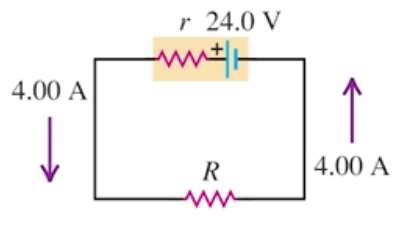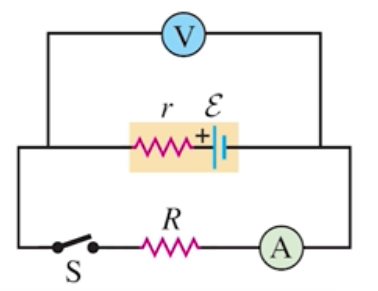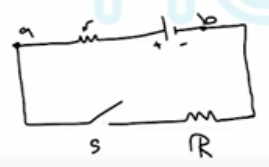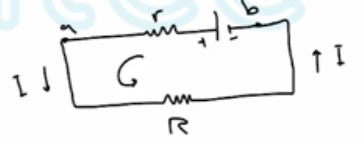Need Help?

Subscribe to Physics 2

###### \${selected_topic_name}
• Notes

$\begin{array}{l}{\text { Consider the circuit shown in Fig. E25.28. The termi- }} \\ {\text { nal voltage of the } 24.0-\mathrm{V} \text { battery is } 21.2 \mathrm{V} . \text { What are (a) the inter- }} \\ {\text { nal resistance } r \text { of the battery and }(\mathrm{b}) \text { the resistance } R \text { of the circuit }} \\ {\text { resistor? }}\end{array}$

$\varepsilon=24 v \quad,\quad I=4 A \quad, \quad V_{a b}=21 \cdot 2 v$

(?) $R??$

(a) $V_{a b}=\varepsilon-I r \Rightarrow r=$$\frac{\varepsilon-V_{a b}}{I}=\frac{24-21 \cdot 2}{4}$

$∴ r=0.7 \Omega$

(b) $V_{a b}-I R=0$$\longrightarrow R=\frac{V_{a b}}{I}=\frac{21.2}{4}=5.3 \Omega$

$R+r=6 \Omega$

$I=\frac{V}{R} \longrightarrow \frac{24}{6}=4 A$

$\begin{array}{l}{\text { A copper transmission cable } 100 \mathrm{km} \text { long and } 10.0 \mathrm{cm} \text { in diameter carries a current of } 125 \mathrm{A} \text { . }} \\ {\text { (a) What is the potential drop across the cable? (b) How much electrical energy is dissipated as }} \\ {\text { thermal energy every hour? }}\end{array}$$R=\frac{\rho L}{A} \quad, \quad V=I R$

$P=V I$                $\text { energy } = P.t$

(a) $\rho=1.72 * 10^{-8} \Omega \cdot m$        $A=\pi r^{2} \quad r=0.05 m$

$R=\frac{\rho L}{A}=\frac{1.72 * 10^{-8} * 100 * 10^{3}}{\pi(0.05)^{2}}=0.219 \Omega$

$V=I R=125 * 0.219=27.4 \mathrm{V}$

(b) $p=V I=27.4 * 125=3422 w=3422 \ \mathrm{J/s}$

$∴ \text { energy } =P t \quad=3422 * 3600=1.23*10^7 J$

$\begin{array}{l}{\text { When switch } \mathrm{S} \text { in Fig. E25.33 }} \\ {\text { is open, the voltmeter } \mathrm{V} \text { of the battery }} \\ {\text { reads } 3.08 \mathrm{V} \text { . When the switch is closed, }} \\ {\text { the voltmeter reading drops to } 2.977 \mathrm{V} \text { , }} \\ {\text { and the ammeter A reads } 1.65 \mathrm{A} \text { . Find }} \\ {\text { the emf, the internal resistance of the }} \\ {\text { battery, and the circuit resistance of the }} \\ {\text { Assume that the two meters are ideal, }} \\ {\text { so they don't affect the circuit. }}\end{array}$Open$I=0$

$V_{a b}=\varepsilon=3.08 V$

Closed$V_{a b}=\varepsilon- I{r}=2.97 V$

$r=\frac{\varepsilon-2.97}{I}$

$=\frac{3 \cdot 08-2.97}{1.65}$$=0.067 \Omega$

$V_{ a b}=I R$$\rightarrow {R}=$$\frac{V_{a b}}{I}$

$=\frac{2.97}{1.65}=1.8 \Omega$